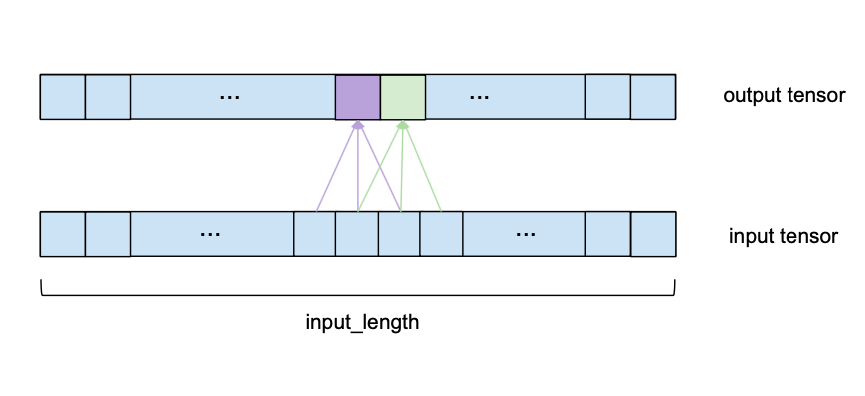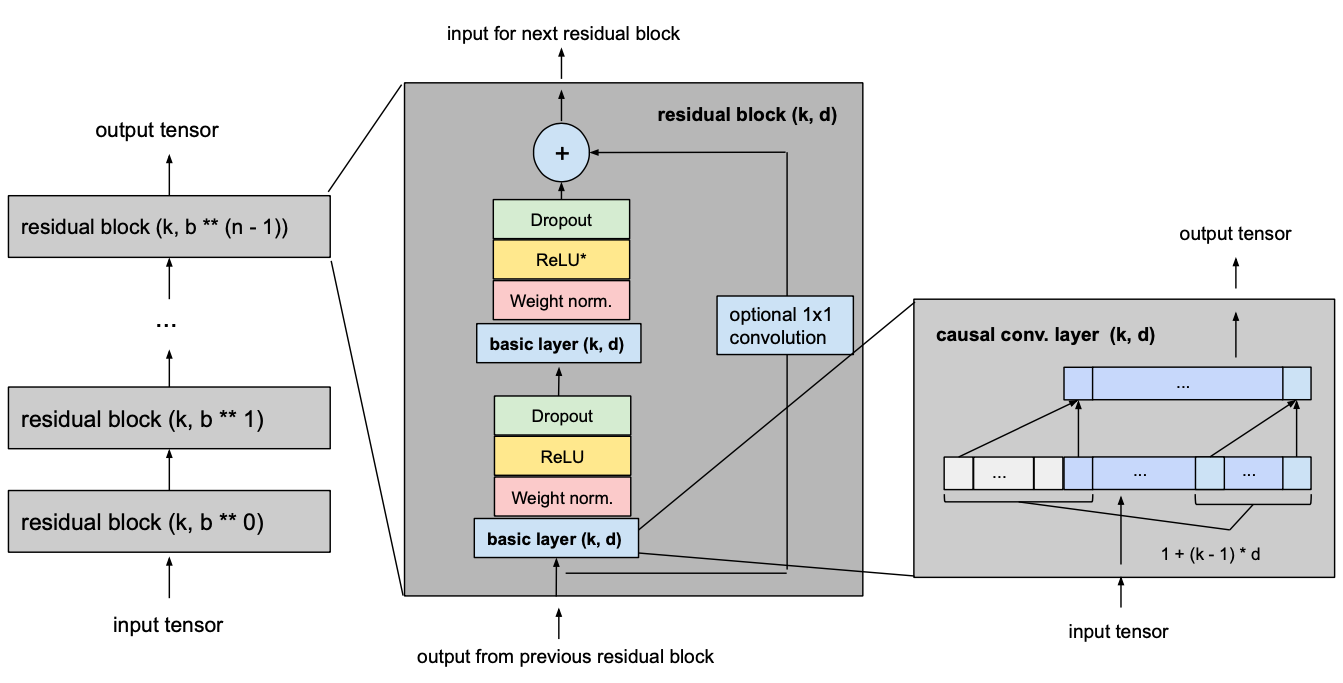## Motivation

Up until recently, the topic of sequence modeling in the context of deep learning has been largely associated with recurrent neural network architectures such as LSTM and GRU. S. Bai et al. (*) suggest that this way of thinking is antiquated, and that convolutional networks should be taken into consideration as one of the primary candidates when modeling sequential data. They were able to show that convolutional networks can achieve better performance than RNNs in many tasks while avoiding common drawbacks of recurrent models, such as the exploding/vanishing gradient problem or lacking memory retention. Furthermore, using a convolutional network instead of a recurrent one can lead to performance improvements as it allows for parallel computation of outputs. The architecture they propose is called Temporal Convolutional Network (TCN) and will be explained in the following sections. To facilitate understanding the TCN architecture in conjunction with its Darts implementation, this article will use the same model parameter names as seen in the library wherever possible (indicated in bold).

## Overview

A TCN, short for Temporal Convolutional Network, consists of dilated, causal 1D convolutional layers with the same input and output lengths. The following sections go into detail about what these terms actually mean.

## 1D Convolutional NetworkWe can see that to compute one element of the output, we look at a series of consecutive elements of length kernel_size of the input. In the above example we chose a kernel_size of 3. To obtain the output, we take the dot product of the subsequence of the input and a kernel vector of learned weights of the same length. To get the next element of the output, the same procedure is applied, but the kernel_size-sized window of the input sequence is shifted to the right by one element (for the purposes of this forecasting model, the stride is always set to 1). Please note that the same set of kernel weights will be used to compute every output of one convolutional layer. The following image shows two consecutive output elements and their respective input subsequences.## Causal Convolution

For a convolutional layer to be causal, for every i in {0, …, input_length — 1} the i’th element of the output sequence may only depend on the elements of the input sequence with indices {0, …, i}. In other words, an element in the output sequence can only depend on elements that come before it in the input sequence. As mentioned before, to ensure an output tensor has the same length as the input tensor, we need to apply zero padding. If we only apply zero-padding on the left side of the input tensor, then causal convolution will be ensured. To understand this, consider the rightmost output element. Given that there is no padding on the right side of the input sequence, the last element it depends on is the last element of the input. Now consider the second to last output element of the output sequence. Its kernel window is shifted to the left by one compared to the last output element, that means its rightmost dependency in the input sequence is the second to last element of the input sequence. It follows by induction that for every element in the output sequence, its latest dependency in the input sequence has the same index as itself. The following figure shows an example with an input_length of 4 and a kernel_size of 3.We can see that with a left zero-padding of 2 entries we can achieve the same output length while obeying the causality rule. In fact, without dilation, the number of zero-padding entries required for maintaining the input length is always equal to kernel_size – 1.

## Dilation

One desirable quality of a forecasting model is that the value of a specific entry in the output depends on all previous entries in the input, i.e. all entries that have an index smaller or equal to itself. This is achieved when the receptive field, meaning the set of entries of the original input that affect a specific entry of the output, has size input_length. We also call this ‘full history coverage’. As we have seen before, one conventional convolutional layer makes an entry in the output dependent on the kernel_size entries of the input that have an index smaller or equal to itself. For instance, if we have a kernel_size of 3, the 5th element in the output will be dependent on elements 3, 4 and 5 of the input. This reach is expanded when we stack multiple layers on top of each other. In the following figure we can see that by stacking two layers with kernel_size 3 we get a receptive field size of 5.More generally, a 1D convolutional network with n layers and a kernel_sizek has a receptive field r of sizeTo know how many layers are needed for full coverage, we can set the receptive field size to input_length l and solve for the number of layers n (we need to round up in case of non-integer values):Here we only show the influence of inputs that affect the last value of the output. Likewise, only zero-padding entries necessary for the last output value are shown. Clearly, the last output value is dependent on the whole input coverage. Actually, given the hyperparameters, an input_length of up to 15 could be used while maintaining full receptive field coverage. Generally speaking, every additional layer adds a value of d*(k-1) to the current receptive field width, where d is computed as d=b**i, with irepresenting the number of layers below our new layer. Consequently, the width of the receptive field w of a TCN with exponential dilation of base b, kernel size k and number of layers n is given byHowever, depending on the values b and k, this receptive field can have ‘holes’. Consider the following network with a dilation_base of 3 and a kernel size of 2:The receptive field does cover a range that is larger than the input size (namely 15). However, the receptive field has holes in it; that is there are entries in the input sequence that the output value is not dependent on (shown above in red). To solve this problem, we need to either increase the kernel size to 3, or decrease the dilation base to 2. Generally speaking, for a receptive field with no holes, the kernel size k has to be at least as big as the dilation base b.

Considering these observations, we can compute how many layers our network needs for full history coverage. Given a kernel size k, dilation base b, where kb, and input length l, the following inequality must hold for full history coverage:We can solve for n and get the minimum number of required layers as## Basic TCN Overview

Given input_length, kernel_size, dilation_base and the minimum number of layers required for full history coverage, the basic TCN network would look something like this:## Forecasting

So far we have only talked about the ‘input sequence’ and the ‘output sequence’ without getting into how they relate to each other. In the context of forecasting, we want to predict the next entries of a time series into the future. To train our TCN network to do forecasting, the training set will consist of (input sequence, target sequence)-pairs of equally-sized subsequences of the given time series. A target series will be a series that is shifted forward in relation to its respective input series by a certain number output_length. This means that a target series of length input_lengthcontains the last (input_length – output_length) elements of its respective input sequence as first elements, and the output_length elements that come after the last entry of the input series as its final elements. In the context of forecasting, this means that the maximum forecasting horizon that can be predicted with such a model is equal to output_length. Using a sliding window approach, many overlapping pairs of input and target sequences can be created out of one time series.## Improvements to the Model

S. Bai et al. (*) suggest a few additions to the basic TCN architecture for improved performance which will be discussed in this section, namely residual connections, regularization and activation functions.

### Residual Blocksbecomeswhich leads to a minimum number of residual blocks n for full history coverage of input_length l of### Activation, Normalization, RegularizationThe asterisk in the second ReLU unit indicates that it is present in every layer but the last one, since we want our final output to be able to take on negative values as well (this differs from the architecture outlined in the paper).

## Final Model

The following picture shows our final TCN model with l equal to input_length, k equal to kernel_size, b equal to dilation_base, kb and with a minimum number of residual blocks for full history coverage n,where n can be computed from the other values as explained above.## Example

```from darts import TimeSeries
from darts.dataprocessing.transformers import
MissingValuesFiller
import pandas as pd

df['time'] = pd.to_datetime(df['time'], utc=True)
df['time']= df.time.dt.tz_localize(None)

df_day_avg =
df.groupby(df['time'].astype(str).str.split(" ").str).mean().reset_index()

value_filler = MissingValuesFiller()
series =
value_filler.transform(TimeSeries.from_dataframe(df_day_avg, 'time', ['generation hydro run-of-river and poundage']))

series.plot()````series = series.add_datetime_attribute('day', one_hot=True)`
```from darts.dataprocessing.transformers import Scaler

train, val =
series.split_after(pd.Timestamp('20170901'))

scaler = Scaler()
train_transformed = scaler.fit_transform(train)
val_transformed = scaler.transform(val)
series_transformed = scaler.transform(series)```
```from darts.models import TCNModel

model = TCNModel(
input_size=train.width,
n_epochs=20,
input_length=365,
output_length=7,
dropout=0,
dilation_base=2,
weight_norm=True,
kernel_size=7,
num_filters=4,
random_state=0
)

model.fit(
training_series=train_transformed,
target_series=train_transformed['0'],
val_training_series=val_transformed,
val_target_series=val_transformed['0'],
verbose=True
)```
```pred_series = model.backtest(
series_transformed,
target_series=series_transformed['0'],
start=pd.Timestamp('20170901'),
forecast_horizon=7,
stride=5,
retrain=False,
verbose=True,
use_full_output_length=True
)```
```from darts.metrics import r2_score
import matplotlib.pyplot as plt

series_transformed[900:]['0'].plot(label='actual')
pred_series.plot(label=('historic 7 day forecasts'))
r2_score_value = r2_score(series_transformed['0'], pred_series)

plt.title('R2:' + str(r2_score_value))
plt.legend()```### Conclusion

Thanks to Julien Herzen.